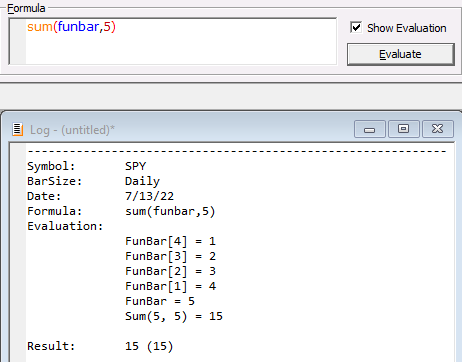Navigation: Realtest Script Language > Syntax Element Details > FunBar

Category

Bar Data Values

Description

Ordinal number of a bar within the the calculation of a multi-bar function.

Notes

FunBar makes it possible, in some cases, to calculate with a one-line function call a complex formula that otherwise need to be written with looping code.

As a simple example of how this works, the following shows the evaluation steps of Sum(FunBar, 5):Within the internal calculation loop of the Sum function, FunBar starts at 1 for the earliest bar, then is incremented as the calculation proceeds.

For a more complex example, see the ehlers_windows.rts example script.